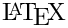Including tables

Doxygen supports two ways to put tables in the documentation.

The easiest is to use the Markdown format as shown in Markdown Extensions section Tables.

Although this format is easy to use and read, it is also rather limited. It supports only a simple grid of cells, while each cell is a single line of plain text.

For more complex tables the HTML syntax can be used. Doxygen will process such tables and translate them to the various output formats (at least for the formats that do support tables such as HTML and).

Note a table should at least contain 1 row (<tr>) and in case a <caption> is used the <caption> should precede the first row.

Here is an example of a complex table:

<table>
<caption id="multi_row">Complex table</caption>
<tr><th>Column 1                      <th>Column 2        <th>Column 3
<tr><td rowspan="2">cell row=1+2,col=1<td>cell row=1,col=2<td>cell row=1,col=3
<tr><td rowspan="2">cell row=2+3,col=2                    <td>cell row=2,col=3
<tr><td>cell row=3,col=1                                  <td rowspan="2">cell row=3+4,col=3
<tr><td colspan="2">cell row=4,col=1+2
<tr><td>cell row=5,col=1              <td colspan="2">cell row=5,col=2+3
<tr><td colspan="2" rowspan="2">cell row=6+7,col=1+2      <td>cell row=6,col=3
<tr>                                                      <td>cell row=7,col=3
<tr><td>cell row=8,col=1              <td>cell row=8,col=2\n
<table>
<tr><td>Inner cell row=1,col=1<td>Inner cell row=1,col=2
<tr><td>Inner cell row=2,col=1<td>Inner cell row=2,col=2
</table>
<td>cell row=8,col=3
<ul>
<li>Item 1
<li>Item 2
</ul>
</table>


It has a caption, table heading, various row and column spans, a nested table as one of the cells, and a item list in another cell.

Note that the end tags (like </td>) are left out in the example above. This is allowed, and in the HTML output doxygen will add the end tags again.

The output will look as follows:

Complex table
Column 1 Column 2 Column 3
cell row=1+2,col=1cell row=1,col=2cell row=1,col=3
cell row=2+3,col=2 cell row=2,col=3
cell row=3,col=1 cell row=3+4,col=3
cell row=4,col=1+2
cell row=5,col=1 cell row=5,col=2+3
cell row=6+7,col=1+2 cell row=6,col=3
cell row=7,col=3
cell row=8,col=1 cell row=8,col=2
 Inner cell row=1,col=1 Inner cell row=1,col=2 Inner cell row=2,col=1 Inner cell row=2,col=2
cell row=8,col=3
• Item 1
• Item 2

One can refer to the caption of the table using \ref using the caption's id as the label.